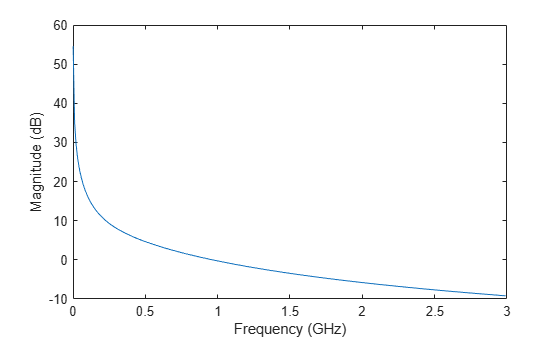Documentation

rfmodel.rational

Store output power and phase information for amplifiers or mixers

Description

Use the rational class to represent RF components using a rational function object of the form:

$F\left(s\right)=\left(\sum _{k=1}^{n}\frac{{C}_{k}}{s-{A}_{k}}+D\right){e}^{-s\tau }\begin{array}{cc},& s=j2\pi f\end{array}$

There are two ways to construct an rational function object:

• You can fit a rational function object to the component data using the rationalfit function.

• You can use the rfmodel.rational constructor to specify the pole-residue representation of the component directly.

Creation

Description

example

h = rfmodel.rational returns a rational function object whose properties are set to their default values.

h = rfmodel.rational('Property1',value1,'Property2',value2,...) sets properties using one or more name-value pairs. You can specify multiple name-value pairs. Enclose each property name in a quote

Properties

expand all

Poles of rational function object, specified as a complex vector in radians/second. The property length is shown in:

$F\left(s\right)=\left(\sum _{k=1}^{n}\frac{{C}_{k}}{s-{A}_{k}}+D\right){e}^{-s\tau }\begin{array}{cc},& s=j2\pi f\end{array}$

where, n must be equal to the length of the vector you provide for 'C'. n is the number of poles in the rational function object. By default, this property is empty.

Data Types: double

Residues of the rational function object, specified as a complex vector in radians/second. The property length is shown in

$F\left(s\right)=\left(\sum _{k=1}^{n}\frac{{C}_{k}}{s-{A}_{k}}+D\right){e}^{-s\tau }\begin{array}{cc},& s=j2\pi f\end{array}$

as n, must be equal to the length of the vector you provide for 'A'. n is the number of residues in the rational function object. By default, this property is empty.

Data Types: double

Frequency response offset, specified as a scalar. The default value is 0.

Data Types: double

Frequency response time delay, specified as a scalar. The default value is 0.

Data Types: double

Object name, specified as a 1-by-N character array. This is a read-only property.

Data Types: char

Object Functions

 freqresp Frequency response of a rational function and rationalfit function object stepresp Step-signal response of rational function object rationalfit Approximate data using stable rational function object ispassive Return true if rationalfit output is passive at all frequencies makepassive Enforce passivity of a rationalfit output passivity Plot passivity of N-by-N rationalfit function output timeresp Time response for rational function object writeva Write Verilog-A description of rational function object generateSPICE Generate SPICE file from rationalfit of S-parameters

Examples

collapse all

Fit a rational function to data from an rfdata.data object.

S = sparameters('defaultbandpass.s2p');
freq = S.Frequencies;
data = rfparam(S,2,1);
fit = rationalfit(freq,data)
fit =
rfmodel.rational with properties:

A: [10x1 double]
C: [10x1 double]
D: 0
Delay: 0
Name: 'Rational Function'

Construct a rational function object, rat, with poles at -4 Mrad/s, -3 Grad/s,and -5 Grad/s and residues of 600 Mrad/s,2 Grad/s and 4 Grad/s.

rat=rfmodel.rational('A',[-5e9,-3e9,-4e6],'C',[6e8,2e9,4e9]);

Perform frequency-domain analysis from 1.0 MHz to 3.0 GHz.

f = [1e6:1.0e7:3e9];

Plot the resulting frequency response in decibels on the X-Y plane.

[resp,freq]=freqresp(rat,f);
figure
plot(freq/1e9,20*log10(abs(resp)));
xlabel('Frequency (GHz)')
ylabel('Magnitude (dB)')Read a file named passive.s2p and fit the 2-by-2 S-parameters. Generate a SPICE file of these S-parameters.

S = sparameters('passive.s2p');
fit = rationalfit(S);
generateSPICE(fit,'passive.ckt')

The circuit is saved in your current folder.